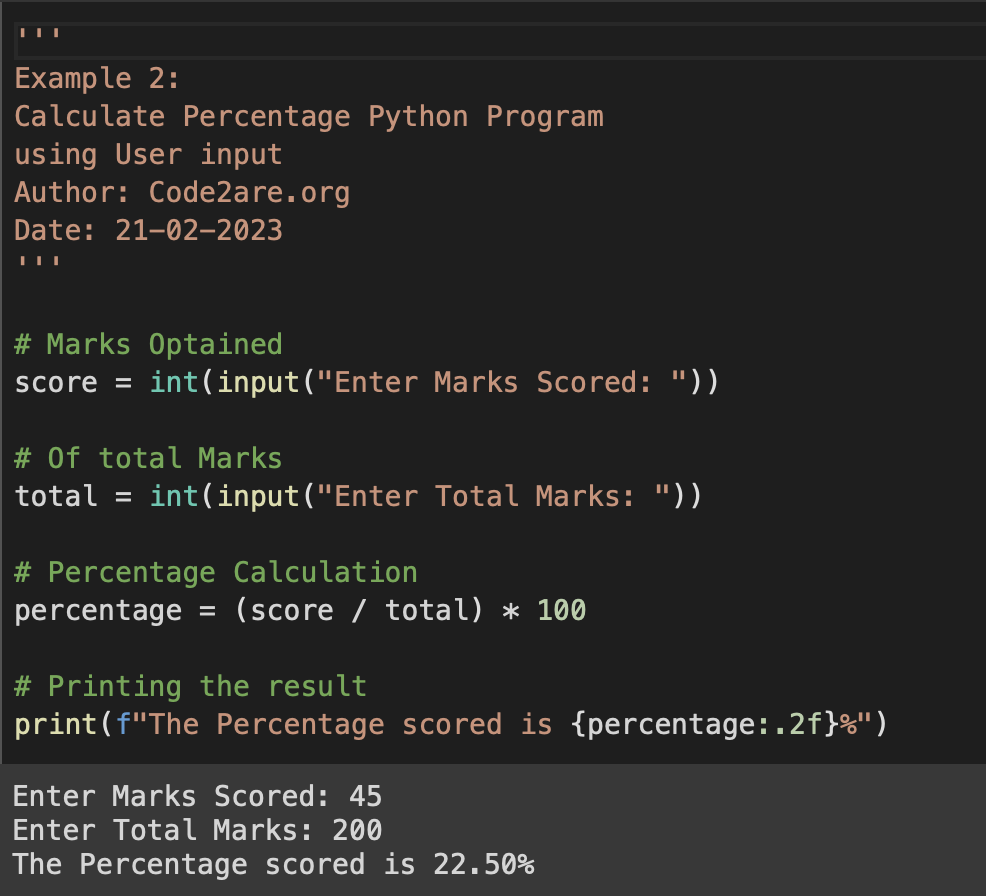# Program 11: Calculate Percentage - 1000+ Python Programs

As a learner, you may get to solve a lot of questions related to percentages in Python Programming in your School or College.

## What is Percentage?

A percentage is a way of expressing a fraction as a portion of 100.

Examples:
• 1/2 can be expressed as a percentage by multiplying it by 100 = 50%
• 4/5 can be expressed as a percentage by multiplying it by 100 = 80%
• 2/3 can be expressed as a percentage by multiplying it by 100 = 66.67%
• 7/10 can be expressed as a percentage by multiplying it by 100 = 70%
• 3/8 can be expressed as a percentage by multiplying it by 100 = 37.5%

Percentages are used in finance, statistics, and other fields to compare numbers relative to a whole.

In Python, you can calculate a percentage by multiplying a number by 100 and dividing it by another number

## Program 1: If a student scored 80 out of 100 on a test, what is their percentage score?

``````# Marks Optained
score = 80

# Of Total Marks
total = 100

# Percentage Calculation
percentage = (score / total) * 100

# Printing the result
print(f"The Percentage scored is {percentage:.2f}%")``````
Output:

The Percentage scored is 80.00%

## Program 2: Calculate Percentage based on user input of marks scored of total marks?

``````'''
Example 2:
Calculate the Percentage of Python Program
using User input
Author: Code2are.org
Date: 21-02-2023
'''

# Marks Obtained
score = int(input("Enter Marks Scored: "))

# Of total Marks
total = int(input("Enter Total Marks: "))

# Percentage Calculation
percentage = (score / total) * 100

# Printing the result
print(f"The Percentage scored is {percentage:.2f}%")``````
Output:

Enter Marks Scored: 45
Enter Total Marks: 200
The Percentage scored is 22.50%-

Have Questions? Post them here!